# Solving Two Step Equations Lesson 4 4 Rules

• Slides: 10Solving Two- Step Equations Lesson 4 -4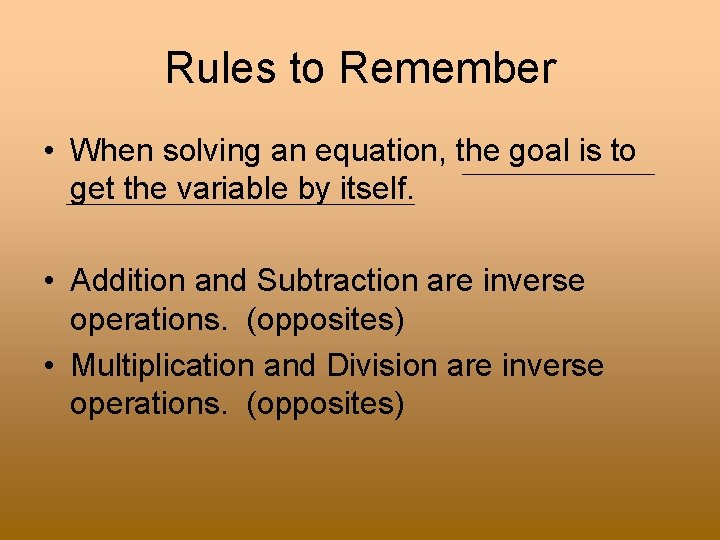Rules to Remember • When solving an equation, the goal is to get the variable by itself. • Addition and Subtraction are inverse operations. (opposites) • Multiplication and Division are inverse operations. (opposites)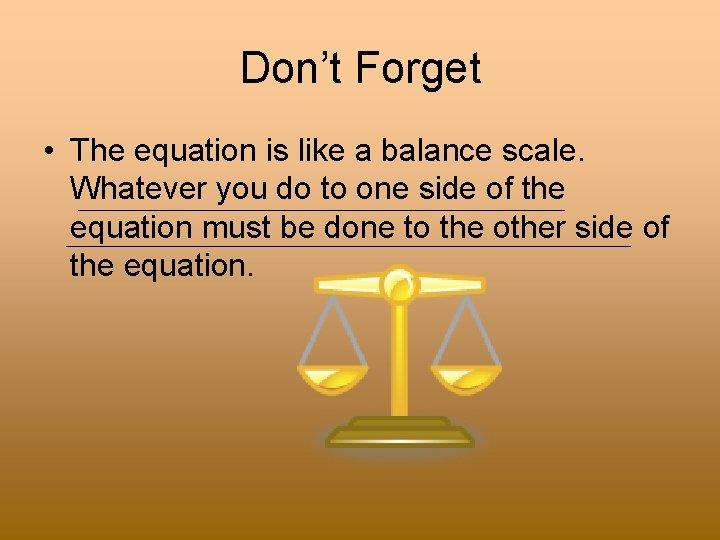Don’t Forget • The equation is like a balance scale. Whatever you do to one side of the equation must be done to the other side of the equation.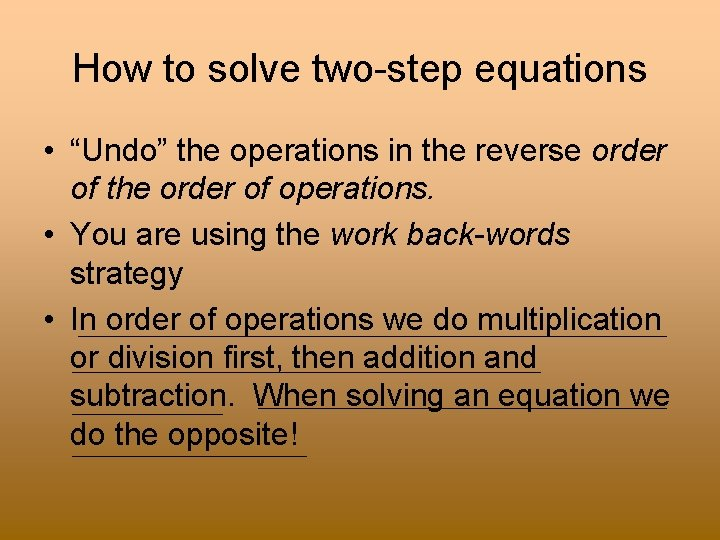How to solve two-step equations • “Undo” the operations in the reverse order of the order of operations. • You are using the work back-words strategy • In order of operations we do multiplication or division first, then addition and subtraction. When solving an equation we do the opposite!Integer Rules to Remember • 1. 2. Adding Integers Same sign= add and keep the sign Different sign = subtract and keep the sign of the larger number Subtracting Integers Keep, Change Follow the rules for addition Multiplying and Dividing Integers Same Sign = Positive Answer Different Sign = Negative Answer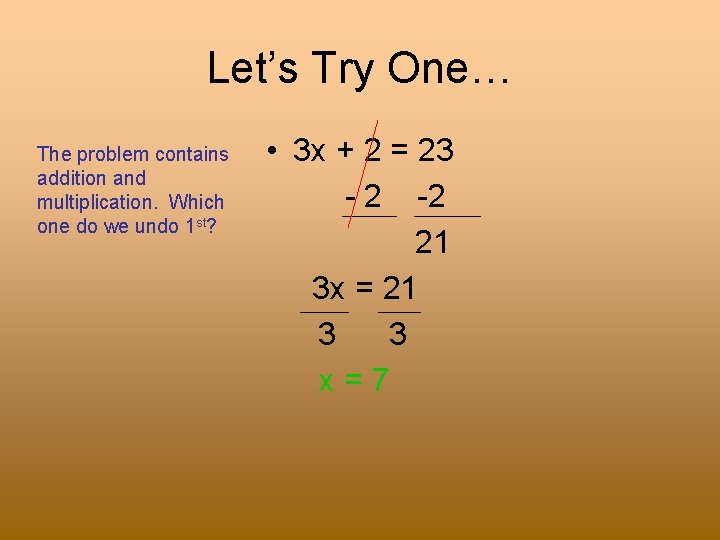Let’s Try One… The problem contains addition and multiplication. Which one do we undo 1 st? • 3 x + 2 = 23 - 2 -2 21 3 x = 21 3 3 x=7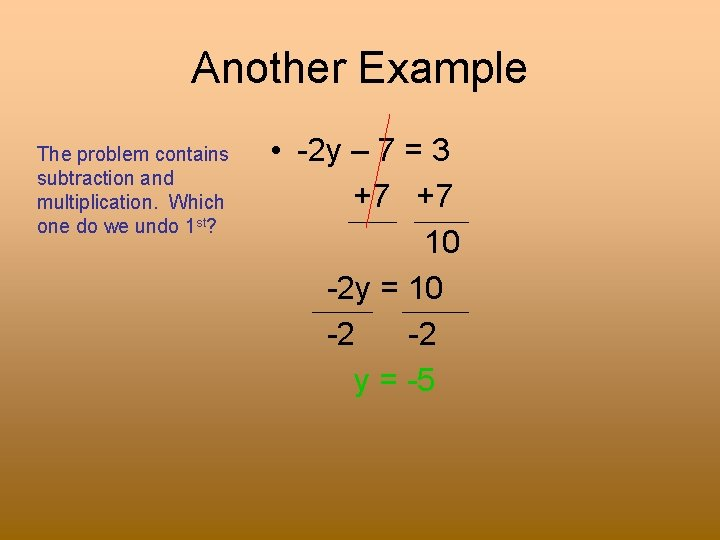Another Example The problem contains subtraction and multiplication. Which one do we undo 1 st? • -2 y – 7 = 3 +7 +7 10 -2 y = 10 -2 -2 y = -5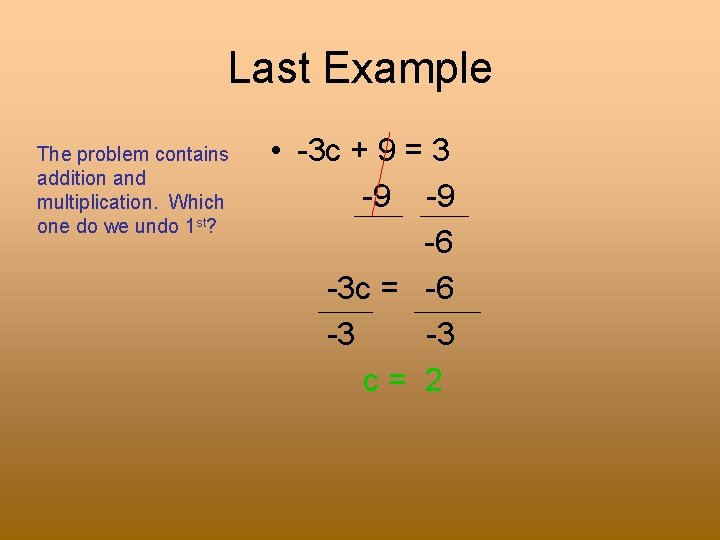Last Example The problem contains addition and multiplication. Which one do we undo 1 st? • -3 c + 9 = 3 -9 -9 -6 -3 c = -6 -3 -3 c= 2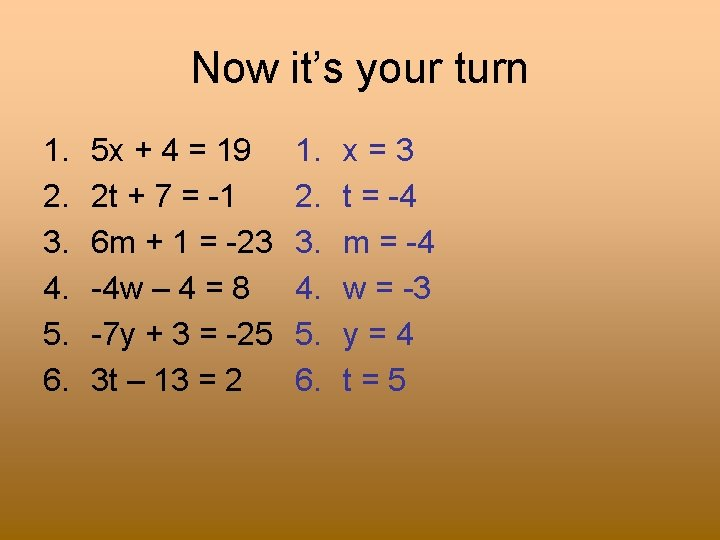Now it’s your turn 1. 2. 3. 4. 5. 6. 5 x + 4 = 19 2 t + 7 = -1 6 m + 1 = -23 -4 w – 4 = 8 -7 y + 3 = -25 3 t – 13 = 2 1. 2. 3. 4. 5. 6. x=3 t = -4 m = -4 w = -3 y=4 t=5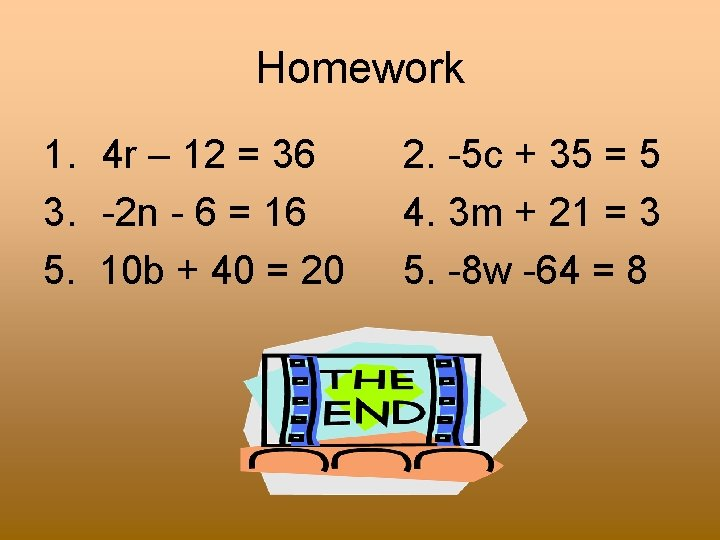Homework 1. 4 r – 12 = 36 3. -2 n - 6 = 16 5. 10 b + 40 = 20 2. -5 c + 35 = 5 4. 3 m + 21 = 3 5. -8 w -64 = 8